SBOS789C August   2017  – February 2020

PRODUCTION DATA.

1. Features
2. Applications
3. Description
1.     Device Images
4. Revision History
5. Device Comparison Table
6. Pin Configuration and Functions
7. Specifications
8. Detailed Description
1. 8.1 Overview
2. 8.2 Functional Block Diagram
3. 8.3 Feature Description
4. 8.4 Device Functional Modes
9. Application and Implementation
1. 9.1 Application Information
2. 9.2 Typical Applications
1. 9.2.1 Transimpedance Amplifier
2. 9.2.2 Multichannel Sensor Interface
10. 10Power Supply Recommendations
11. 11Layout
1. 11.1 Layout Guidelines
2. 11.2 Layout Example
12. 12Device and Documentation Support
13. 13Mechanical, Packaging, and Orderable Information

• D|8
• DCN|8
• DGK|8
• DCN|8
• DGK|8

#### 9.1.1 Selection of Feedback Resistors

The OPA2810 is a classic voltage feedback amplifier with each channel having two high-impedance inputs and a low-impedance output. Standard application circuits include the noninverting and inverting gain configurations as Figure 64 and Figure 65 show. The DC operating point for each configuration is level-shifted by the reference voltage VREF which is typically set to midsupply in single-supply operation. VREF is often connected to ground in split-supply applications.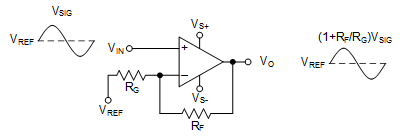Figure 64. Noninverting Amplifier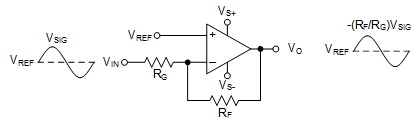Figure 65. Inverting Amplifier

The closed-loop gain of an amplifier in noninverting configuration is shown in Equation 1.

Equation 1.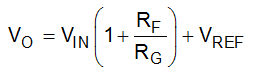The closed-loop gain of an amplifier in an inverting configuration is shown in Equation 2.

Equation 2.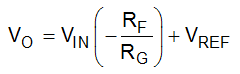The magnitude of the low-frequency gain is determined by the ratio of the magnitudes of the feedback resistor (RF) and the gain setting resistor RG. The order of magnitudes of the individual values of RF and RG offer a trade-off between amplifier stability, power dissipated in the feedback resistor network, and total output noise. The feedback network increases the loading on the amplifier output. Using large values of the feedback resistors reduces the power dissipated at the amplifier output. On the other hand, this increases the inherent voltage and amplifier current noise contribution seen at the output while lowering the frequency at which a pole occurs in the feedback factor (β). This pole causes a decrease in the phase margin at zero-gain crossover frequency and potential instability. Using small feedback resistors increases power dissipation and also degrades amplifier linearity due to a heavier amplifier output load. Figure 66 shows a representative schematic of the OPA2810 in an inverting configuration with the input capacitors shown.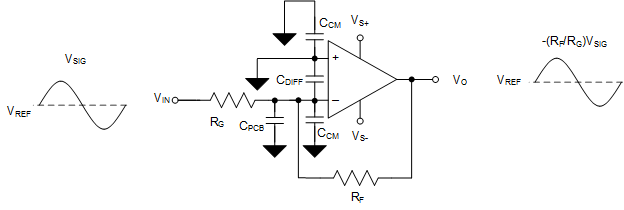Figure 66. Inverting Amplifier with Input Capacitors

The effective capacitance seen at the amplifier's inverting input pin is shown in Equation 3 which forms a pole in β at a cut-off frequency of Equation 4.

Equation 3.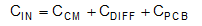Equation 4.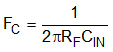where:

• CCM is the amplifier common-mode input capacitance
• CDIFF is the amplifier differential input capacitance
• and, CPCB is the PCB parasitic capacitance.

For low-power systems, greater the values of the feedback resistors, the earlier in frequency does the phase margin begin to reduce and cause instability. Figure 67 and Figure 68 illustrate the loop gain magnitude and phase plots, respectively, for the OPA2810 simulation in TINA-TI configured as an inverting amplifier with values of feedback resistors varying by orders of magnitudes.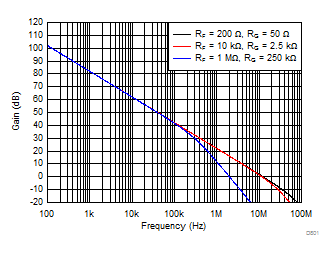Figure 67. Loop-Gain vs. Frequency for Circuit of Figure 66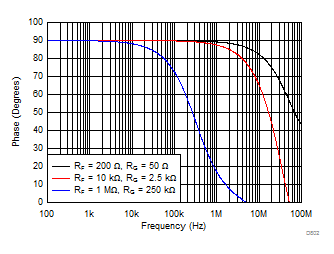Figure 68. Loop-Gain Phase vs. Frequency for Circuit of Figure 66

A lower phase margin results in peaking in the frequency response and lower bandwidth as Figure 69 shows, which is synonymous with overshoot and ringing in the pulse response results. The OPA2810 offers a flat-band voltage noise density of 6 nV/√Hz. TI recommends selecting an RF so the voltage noise contribution does not exceed that of the amplifier. Figure 70 shows the voltage noise density variation with value of resistance at 25°C. A 2-kΩ resistor exhibits a thermal noise density of 5.75 nV/√Hz which is comparable to the flatband noise of the OPA2810. Hence, TI recommends using an RF lower than 2 kΩ while being large enough to not dissipate excessive power for the output voltage swing and supply current requirements of the application. The Noise Analysis and the Effect of Resistor Elements on Total Noise section shows a detailed analysis of the various contributors to noise.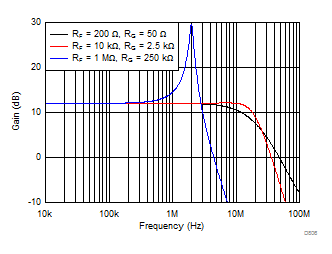Figure 69. Closed-Loop Gain vs. Frequency for Circuit of Figure 66Figure 70. Thermal Noise Density vs Resistance